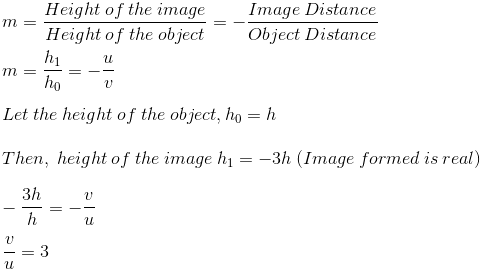• +91 9971497814
• info@interviewmaterial.com

# Chapter 10- Light-Reflection and Refraction Interview Questions Answers

### Related Subjects

Question 1 : Define the principal focus of a concave mirror.

Answer 1 : Light rays that are parallel to the principal axis of a concave mirror converge at a specific point on its principal axis after reflecting from the mirror. This point is called the principal focus of the concave mirror.

Question 2 : The radius of curvature of a spherical mirror is 20 cm. What is its focal length?

Radius of curvature (R) = 20 cm
Radius of curvature of the spherical mirror = 2 × Focal length (f)
R = 2f
f= R/2 = 20 / 2 = 10
Therefore, the focal length of the spherical mirror is 10 cm.

Question 3 : Name the mirror that can give an erect and enlarged image of an object.

Answer 3 : The mirror that can give an erect and enlarged image of an object is Concave Mirror.

Question 4 : Why do we prefer a convex mirror as a rear-view mirror in vehicles?

Answer 4 : Convex mirror is preferred as a rear-view mirror in cars and vehicles as it gives a wider field of view, which helps the driver to see most of the traffic behind him. Convex mirrors always form an erect, virtual, and diminished image of the objects placed in front of it.

Question 5 : Find the focal length of a convex mirror whose radius of curvature is 32 cm.

Radius of curvature (R) = 32 cm
Radius of curvature = 2 × Focal length (f)
R= 2f
f = R/2 = 32/2 = 16
Therefore, the focal length of the given convex mirror is 16 cm.

Question 6 : A concave mirror produces three times magnified (enlarged) real image of an object placed at 10 cm in front of it. Where is the image located?

Answer 6 : Magnification produced by a spherical mirror:Object distance (u) = – 10 cm
v = 3 × (- 10) = – 30 cm
Therefore, the negative sign indicates that an inverted image is formed in front of the given concave mirror at a distance of 30 cm.

Question 7 : A ray of light travelling in air enters obliquely into water. Does the light ray bends towards the normal or away from the normal? Why?

Answer 7 : The light ray bends towards the normal. When a light ray enters from an optically rarer medium (which has low refractive index) to an optically denser medium (which has a high refractive index), its speed slows down and bends towards the normal. As water is optically denser than air, a ray of light entering from air into water will bend towards the normal.

Question 8 : Light enters from air to glass having refractive index 1.50. What is the speed of light in the glass? The speed of light in vacuum is 3 x 108 ms-1.

Refractive index of a medium (nm) = Speed of light in vacuum/Speed of light in the medium
Speed of light in vacuum (c) = 3 × 108 m/s
Refractive index of glass (ng) = 1.50
Speed of light in the glass (v) = Speed of light in vacuum/ Refractive index of glass
= c/ng
=3 × 108/1.50 = 2x 108 ms-1.

Question 9 : Find out, from Table, the medium having highest optical density. Also find the medium with lowest optical density.

 Material medium Refractive index Material medium Refractive index Air 1.0003 Canada Balsam 1.53 Ice 1.31 – – Water 1.33 Rock salt 1.54 Alcohol 1.36 – – Kerosene 1.44 Carbon disulphide 1.63 Fused quartz 1.46 Dense flint glass 1.65 Turpentine oil 1.47 Ruby 1.71 Benzene 1.50 Sapphire 1.77 Crown glass 1.52 Diamond 2.42

Lowest optical density = Air
Highest optical density = Diamond
The optical density of a medium is directly related to its refractive index. A medium with the highest refractive index will have the highest optical density and vice-versa.
It can be observed from the table that air and diamond respectively have the lowest and highest refractive index. Hence, air has the lowest optical density and diamond has the highest optical density.

Question 10 : You are given kerosene, turpentine and water. In which of these does the light travel fastest? Use the information given in Table.

 Material medium Refractive index Material medium Refractive index Air 1.0003 Canada Balsam 1.53 Ice 1.31 – – Water 1.33 Rock salt 1.54 Alcohol 1.36 – – Kerosene 1.44 Carbon disulphide 1.63 Fused quartz 1.46 Dense flint glass 1.65 Turpentine oil 1.47 Ruby 1.71 Benzene 1.50 Sapphire 1.77 Crown glass 1.52 Diamond 2.42
Light travel faster in water as compared to kerosene & turpentine as the refractive index of water is lower than that of kerosene and turpentine. The speed of light is inversely proportional to the refractive index.

Todays Deals### Chapter 10- Light-Reflection and Refraction Contributorskrishan

Name:
Email:

# Latest News# 9000 interview questions in different categories